# Test: Sequential Logic Circuits

## 10 Questions MCQ Test Topicwise Question Bank for Electronics Engineering | Test: Sequential Logic Circuits

Description
Attempt Test: Sequential Logic Circuits | 10 questions in 30 minutes | Mock test for GATE preparation | Free important questions MCQ to study Topicwise Question Bank for Electronics Engineering for GATE Exam | Download free PDF with solutions
QUESTION: 1

### For which of the following flip-flops, the output is clearly defined for all combinations of two inputs?

Solution:

For J-K flip-flop, the output is clearly defined for all combinations of two inputs. For S-R flip-flop output is not defined when S = R = 1. A D flip-flop has only one input.

QUESTION: 2

### What J-K input condition will always set ‘Q+ upon the occurrence of the active clock transition ?

Solution:

Truth table for J-K flip-flop is shown below.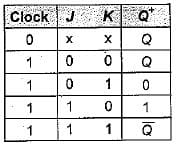When J = 1 and K = 0, output (Q+) is always set upon the occurrence of the active clock transition.

QUESTION: 3

### In a J-K flip-flip, toggle means

Solution:

In a J-K flip-flop, toggle means change the output to the opposite state. A J-K flip-flop toggles when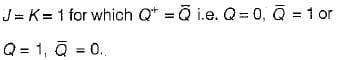QUESTION: 4

The output of S-R flip-flop when S = 1, R = 0 is

Solution: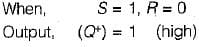QUESTION: 5

An eight stage ripple counter uses a flip-flop with propagation delay of 75 nano-seconds. The pulse width of the strobe is 50 nano-seconds. The frequency of the input signal which can be used for proper operation of the counter is approximately equal to

Solution:

Maximum time taken for all flip-flops to stabilize is (75 ns x 8) + 50 ns = 650 ns.
Frequency of operation must be less than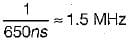Thus, option (d) is correct.

QUESTION: 6

The output of a J-K flip-flop with asynchronous preset and clear inputs if ‘1 ’. The output can be changed to ‘0’ with which one of the following conditions?

Solution:

When J = 1, K = 1 and the clock, next state will be complement of the present state. Thus,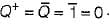QUESTION: 7

A J-K flip-flop can be implemented using D flip- flop connected such that

Solution:
QUESTION: 8

Assertion (A): In general, asynchronous circuits are considerably faster than synchronous circuits.
Reason (R): In an asynchronous circuit, events can occur after one event is completed and there is no need to wait for a clock pulse.

Solution:
QUESTION: 9

Consider the following statements:
1. Sequential circuits are always faster than combination circuits.
2. In an asynchronous circuit there is no problem of stability.
3. The logic circuits whose outputs at any instant of time depend only on the input signals present at that time are known as combinational circuits.
4. In a combinational circuit, for a change in the input, the output appears immediately.
5. In a sequential circuits, the output signals are fed back to the input side.

Which of the statement given above are correct?

Solution:

• Combinational circuits are often faster than sequential circuits since the combinations circuits do not require memory whereas the sequential circuits need memory devices to perform their operations in sequence. Hence statement - 1 is not correct.
• in an asynchronous circuit, events are allowed to occur without any synchronisation In such a case, the system become: unstable which results in difficulties. Hence statement-2 is not correct.
• Statement-3 is correct which is the definitioi of a combinational circuit.
• in a combinational circuit, for a change if the input, the output appears immediately except for the propagation delay througt circuit gates. Thus, statement-4 is no correct.
• In a sequential circuit, an output signal is e function of the present input signals and e sequence of the past input signals i.e. the past output signals since the output signals are fed back to the input side. Hence, statement-5 is correct.
Thus, statements 3 and 5 are only correct.

QUESTION: 10

Assertion (A): A latch is a memory device with the capability of storing one binary digit of information.
Reason (R): A basic latch is made up of cross coupled inverters.

Solution:

A latch is memory device with the capability of storing one binary digit of information because the latch output will remain set/reset until the trigger pulse is given to change the state.
A basic latch is made up of cross coupled inverters as shown below.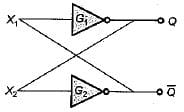Thus, both assertion and reason are true but reason is not the correct explanation of assertion.Use Code STAYHOME200 and get INR 200 additional OFF Use Coupon Code